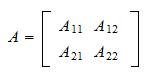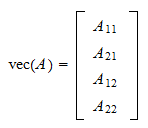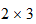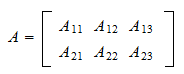StatLect

# Random matrix

A matrix whose entries are random variables is called random matrix.## Associated random vector

A random matrix is usually dealt with by studying and specifying the characteristics of the associated random vector, obtained by stacking its columns.

For example, given arandom matrixwe can study its associated vectorizationwhich is a random vector and can be dealt with using all the standard tools usually employed to deal with random vectors.

Another example is arandom matrixwhose vectorization isThus, no separate theory is developed to deal with random matrices, and all the theory developed for random vectors applies to random matrices.

For example, a random matrix is said to be discrete if the associated random vector is discrete and it is said to be continuous if the associated random vector is continuous.

## The most important matrix distribution

There is a random martrix whose distribution is very important and is used in various places in probability and statistics. It is the Wishart distribution, which has many interesting connections with other distributions such as the Gamma and the multivariate normal. You are referred to the lecture on the Wishart distribution for more details.

## More details

The lecture entitled Random vectors provides more details about random matrices and their probability distributions.